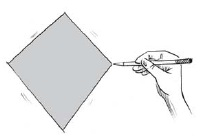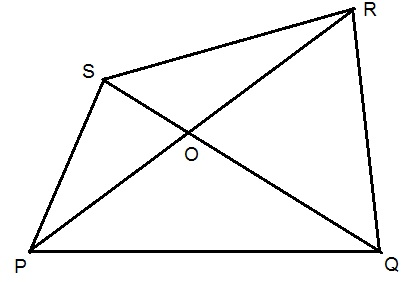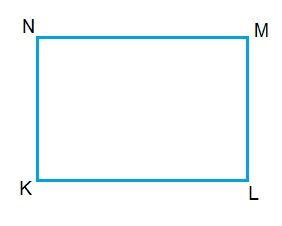Study Materials

# NCERT Solutions for Class 6th Mathematics

Page 5 of 6

## Chapter 4. Basic Geometrical Ideas

### Exercise 4.5

Exercise 4.5

1. Draw a rough sketch of a quadrilateral PQRS. Draw its diagonals. Name them. Is the meeting point of the diagonals in the interior or exterior of the quadrilateral?Solution:Diagonals of quadrilateral PQRS are PR and QS

Meeting Point is O which is interior of quadrilateral PQRS.

2. Draw a rough sketch of a quadrilateral KLMN. State,
(a) two pairs of opposite sides,
(b) two pairs of opposite angles,
(c) two pairs of adjacent sides,
(d) two pairs of adjacent angles.

Solution:(a) two pairs of opposite sides,

Solution:

(i) KL and NM

(ii) KN and LM

(b) two pairs of opposite angles,

Solution:

(i) ∠K and ∠M

(ii) ∠L and ∠N
(c) two pairs of adjacent sides,

Solution:

(i) KN and KL

(ii) KL and ML

(d) two pairs of adjacent angles.

Solution:

(i) ∠K and ∠L

(ii) ∠M and ∠N

Page 5 of 6

Chapter Contents: